## Percent

Percentage Basics:

Centum means hundred, cent is an abbreviation for centum. Therefore,

Cent means hundred. Per cent means per 100.

The symbol for denoting percent is %.

Per cent which is per 100 means something divided by 100 or something out of 100.

In clearer words, percent is a fraction with denominator 100

Therefore, 50 percent means 50 per hundred and it is denoted as 50%.

As a fraction, 50% is written as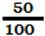which is the fraction

Percent is a fraction with 100 for denominator.

Consider the following examples:

30/100 = 30%, 75/100 = 75%,

100/100 = 100%, 200/100 = 200%,

250% = 250/100 ,  1000% = 1000/100

2.2/100 = 2.2%, 33.54/100 = 33.54%,

Very Important Note:

Write 50th % as a fraction?

First recall, how you write 50% as a fraction.

It is 50/100.

Stretch your imagination and think of all possibilities as to what could this 50th be!

Now, number 50th is the fraction 1/50.

In 50th %, write 1/50 for 50th and the fraction 1/100 for %

Therefore,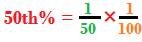Alternative for understanding percentages:

Consider a few percent examples converted into fractions: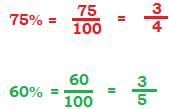2. How to find percentage of a number?

1. If you scored 90% of max 150 in a math test, then you would get

2. Suppose your income is 6, 00,000 and if you saved 30% of your income every month, your monthly savings would be

What did you notice in the above two examples?

The operation × was written for “of”.

Examples:

1. 50% of 60 = 50% ×60 = (50/100) ×60 = 30

2. 30% of 90 = 30%×90 = (30/100) × 90 = 30

Remember the following formula to find percentage of a number:

What is x percent of y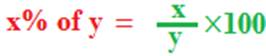1. 40% of a class having 80 students like coffee. How many like coffee?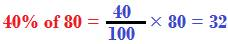2. In the above question, how many do not like coffee?

If 40% like coffee, then the remaining 60% do not like coffee.

And the number is:

Note:

The smarter way to find how many do not like coffee is

80 – 32 = 48

Some more percent examples: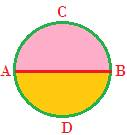In the above figure, area of the circle is 100. AB is diameter of the circle. What percent of the circle is area of the segment ACB?

(Area of segment ACB)/ (area of circle) = 50/100

But, 50/100 = 50 × (1/100) = 50%, because 1/100 is same as %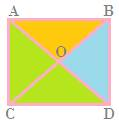Area of the square ABDC above is 100 sq cm. O is mid-point of the diagonals. What percent of the square is

Since O is mid-point of the diagonals, therefore, each of the 4 triangles are equal in area.

Area of each green triangle is 25 sq.cm, so, area of the two green triangles is 50 sq.cm.

Percentage of the area of the two green triangles out of the square is

50/100 = 50%

25/100 = 25%

What did you notice in the above two examples?

What would you write for the question?

What % is A of B?

Mnemonic:

Write what follows “of” in denominator and what follows “is” in numerator.Example:

What % of 25 is 12.5?

Write 12.5 in numerator as it follows “is” and 25 in denominator that follows “of”

So, we have

12.5/25)

Percentage lessons:

Lesson no. 1

1. Examples explaining percentage
2. What % of A is B? or What % is B of A?
3. What % of B is A? Or what % is A of B?

Lesson No. 2

Convert

Lesson no. 3

Word problems in percentages with units